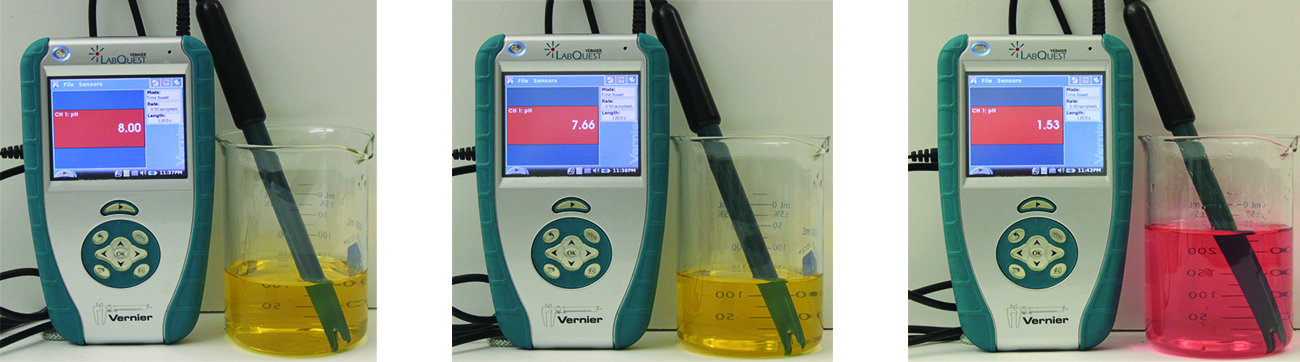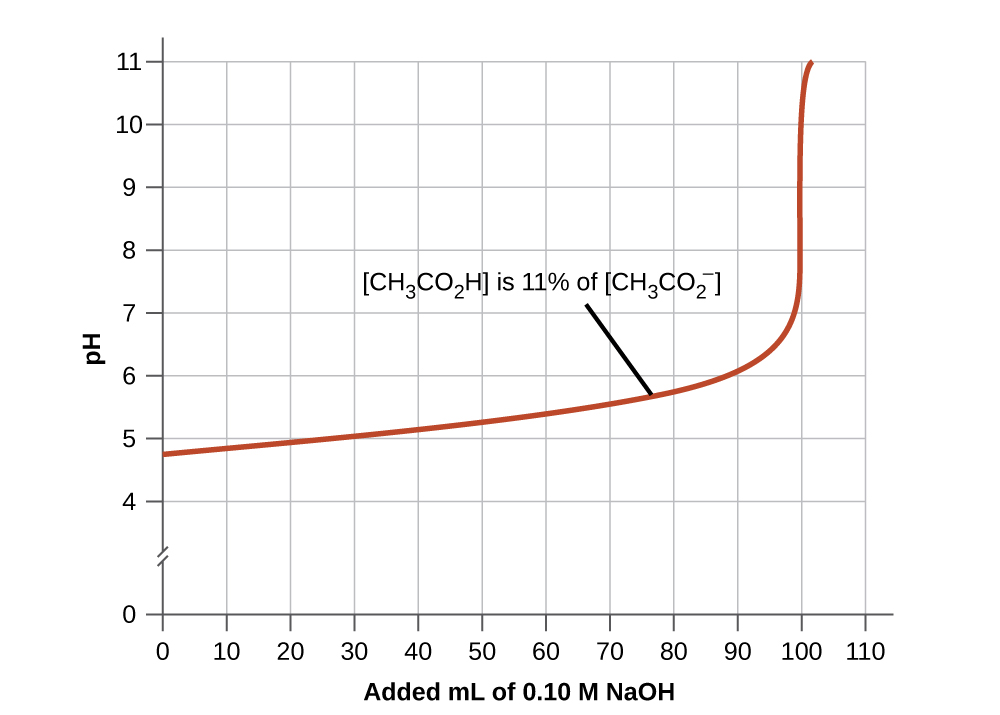# 8.6 Buffers  (Page 3/9)

 Page 3 / 9
$\left(1.0\phantom{\rule{0.2em}{0ex}}×\phantom{\rule{0.2em}{0ex}}{10}^{-4}\right)\phantom{\rule{0.2em}{0ex}}-\phantom{\rule{0.2em}{0ex}}\left(1.8\phantom{\rule{0.2em}{0ex}}×\phantom{\rule{0.2em}{0ex}}{10}^{-6}\right)\phantom{\rule{0.2em}{0ex}}=9.8\phantom{\rule{0.2em}{0ex}}×\phantom{\rule{0.2em}{0ex}}{10}^{-5}\phantom{\rule{0.2em}{0ex}}M$

The concentration of NaOH is:

$\frac{9.8\phantom{\rule{0.2em}{0ex}}×\phantom{\rule{0.2em}{0ex}}{10}^{-5}\phantom{\rule{0.2em}{0ex}}M\phantom{\rule{0.2em}{0ex}}\text{NaOH}}{0.101\phantom{\rule{0.2em}{0ex}}\text{L}}\phantom{\rule{0.2em}{0ex}}=9.7\phantom{\rule{0.2em}{0ex}}×\phantom{\rule{0.2em}{0ex}}{10}^{-4}M$

The pOH of this solution is:

$\text{pOH}=\text{−log}\left[{\text{OH}}^{\text{−}}\right]\phantom{\rule{0.2em}{0ex}}=\text{−log}\left(9.7\phantom{\rule{0.2em}{0ex}}×\phantom{\rule{0.2em}{0ex}}{10}^{-4}\right)\phantom{\rule{0.2em}{0ex}}=3.01$

The pH is:

$\text{pH}=14.00\phantom{\rule{0.2em}{0ex}}-\phantom{\rule{0.2em}{0ex}}\text{pOH}=10.99$

The pH changes from 4.74 to 10.99 in this unbuffered solution. This compares to the change of 4.74 to 4.75 that occurred when the same amount of NaOH was added to the buffered solution described in part (b).

Show that adding 1.0 mL of 0.10 M HCl changes the pH of 100 mL of a 1.8 $×$ 10 −5 M HCl solution from 4.74 to 3.00.

Initial pH of 1.8 $×$ 10 −5 M HCl; pH = −log[H 3 O + ] = −log[1.8 $×$ 10 −5 ] = 4.74
Moles of H 3 O + in 100 mL 1.8 $×$ 10 −5 M HCl; 1.8 $×$ 10 −5 moles/L $×$ 0.100 L = 1.8 $×$ 10 −6
Moles of H 3 O + added by addition of 1.0 mL of 0.10 M HCl: 0.10 moles/L $×$ 0.0010 L = 1.0 $×$ 10 −4 moles; final pH after addition of 1.0 mL of 0.10 M HCl:

$\text{pH}=\text{−log}\left[{\text{H}}_{3}{\text{O}}^{\text{+}}\right]=\text{−log}\left(\phantom{\rule{0.2em}{0ex}}\frac{\text{total moles}\phantom{\rule{0.2em}{0ex}}{\text{H}}_{3}{\text{O}}^{\text{+}}}{\text{total volume}}\right)\phantom{\rule{0.2em}{0ex}}\phantom{\rule{0.2em}{0ex}}=\text{−log}\left(\phantom{\rule{0.2em}{0ex}}\frac{1.0\phantom{\rule{0.2em}{0ex}}×\phantom{\rule{0.2em}{0ex}}{10}^{-4}\phantom{\rule{0.2em}{0ex}}\text{mol}+1.8\phantom{\rule{0.2em}{0ex}}×\phantom{\rule{0.2em}{0ex}}{10}^{-6}\phantom{\rule{0.2em}{0ex}}\text{mol}}{101\phantom{\rule{0.2em}{0ex}}\text{mL}\left(\phantom{\rule{0.2em}{0ex}}\frac{1\phantom{\rule{0.2em}{0ex}}\text{L}}{1000\phantom{\rule{0.2em}{0ex}}\text{mL}}\right)\phantom{\rule{0.2em}{0ex}}}\right)\phantom{\rule{0.2em}{0ex}}\phantom{\rule{0.2em}{0ex}}=3.00$

If we add an acid or a base to a buffer that is a mixture of a weak base and its salt, the calculations of the changes in pH are analogous to those for a buffer mixture of a weak acid and its salt.

## Buffer capacity

Buffer solutions do not have an unlimited capacity to keep the pH relatively constant ( [link] ). If we add so much base to a buffer that the weak acid is exhausted, no more buffering action toward the base is possible. On the other hand, if we add an excess of acid, the weak base would be exhausted, and no more buffering action toward any additional acid would be possible. In fact, we do not even need to exhaust all of the acid or base in a buffer to overwhelm it; its buffering action will diminish rapidly as a given component nears depletion.The indicator color (methyl orange) shows that a small amount of acid added to a buffered solution of pH 8 (beaker on the left) has little affect on the buffered system (middle beaker). However, a large amount of acid exhausts the buffering capacity of the solution and the pH changes dramatically (beaker on the right). (credit: modification of work by Mark Ott)

The buffer capacity    is the amount of acid or base that can be added to a given volume of a buffer solution before the pH changes significantly, usually by one unit. Buffer capacity depends on the amounts of the weak acid and its conjugate base that are in a buffer mixture. For example, 1 L of a solution that is 1.0 M in acetic acid and 1.0 M in sodium acetate has a greater buffer capacity than 1 L of a solution that is 0.10 M in acetic acid and 0.10 M in sodium acetate even though both solutions have the same pH. The first solution has more buffer capacity because it contains more acetic acid and acetate ion.

## Selection of suitable buffer mixtures

There are two useful rules of thumb for selecting buffer mixtures:

1. A good buffer mixture should have about equal concentrations of both of its components. A buffer solution has generally lost its usefulness when one component of the buffer pair is less than about 10% of the other. [link] shows an acetic acid-acetate ion buffer as base is added. The initial pH is 4.74. A change of 1 pH unit occurs when the acetic acid concentration is reduced to 11% of the acetate ion concentration.The graph, an illustration of buffering action, shows change of pH as an increasing amount of a 0.10- M NaOH solution is added to 100 mL of a buffer solution in which, initially, [CH 3 CO 2 H] = 0.10 M and [ CH 3 CO 2 − ] = 0.10 M .
2. Weak acids and their salts are better as buffers for pHs less than 7; weak bases and their salts are better as buffers for pHs greater than 7.

where we get a research paper on Nano chemistry....?
nanopartical of organic/inorganic / physical chemistry , pdf / thesis / review
Ali
what are the products of Nano chemistry?
There are lots of products of nano chemistry... Like nano coatings.....carbon fiber.. And lots of others..
learn
Even nanotechnology is pretty much all about chemistry... Its the chemistry on quantum or atomic level
learn
da
no nanotechnology is also a part of physics and maths it requires angle formulas and some pressure regarding concepts
Bhagvanji
hey
Giriraj
Preparation and Applications of Nanomaterial for Drug Delivery
revolt
da
Application of nanotechnology in medicine
what is variations in raman spectra for nanomaterials
ya I also want to know the raman spectra
Bhagvanji
I only see partial conversation and what's the question here!
what about nanotechnology for water purification
please someone correct me if I'm wrong but I think one can use nanoparticles, specially silver nanoparticles for water treatment.
Damian
yes that's correct
Professor
I think
Professor
Nasa has use it in the 60's, copper as water purification in the moon travel.
Alexandre
nanocopper obvius
Alexandre
what is the stm
is there industrial application of fullrenes. What is the method to prepare fullrene on large scale.?
Rafiq
industrial application...? mmm I think on the medical side as drug carrier, but you should go deeper on your research, I may be wrong
Damian
How we are making nano material?
what is a peer
What is meant by 'nano scale'?
What is STMs full form?
LITNING
scanning tunneling microscope
Sahil
how nano science is used for hydrophobicity
Santosh
Do u think that Graphene and Fullrene fiber can be used to make Air Plane body structure the lightest and strongest. Rafiq
Rafiq
what is differents between GO and RGO?
Mahi
what is simplest way to understand the applications of nano robots used to detect the cancer affected cell of human body.? How this robot is carried to required site of body cell.? what will be the carrier material and how can be detected that correct delivery of drug is done Rafiq
Rafiq
if virus is killing to make ARTIFICIAL DNA OF GRAPHENE FOR KILLED THE VIRUS .THIS IS OUR ASSUMPTION
Anam
analytical skills graphene is prepared to kill any type viruses .
Anam
Any one who tell me about Preparation and application of Nanomaterial for drug Delivery
Hafiz
what is Nano technology ?
write examples of Nano molecule?
Bob
The nanotechnology is as new science, to scale nanometric
brayan
nanotechnology is the study, desing, synthesis, manipulation and application of materials and functional systems through control of matter at nanoscale
Damian
Is there any normative that regulates the use of silver nanoparticles?
what king of growth are you checking .?
Renato
What fields keep nano created devices from performing or assimulating ? Magnetic fields ? Are do they assimilate ?
why we need to study biomolecules, molecular biology in nanotechnology?
?
Kyle
yes I'm doing my masters in nanotechnology, we are being studying all these domains as well..
why?
what school?
Kyle
biomolecules are e building blocks of every organics and inorganic materials.
Joe
how do you find theWhat are the wavelengths and energies per photon of two lines
The eyes of some reptiles are sensitive to 850 nm light. If the minimum energy to trigger the receptor at this wavelength is 3.15 x 10-14 J, what is the minimum number of 850 nm photons that must hit the receptor in order for it to be triggered?
A teaspoon of the carbohydrate sucrose contains 16 calories, what is the mass of one teaspoo of sucrose if the average number of calories for carbohydrate is 4.1 calories/g?
4. On the basis of dipole moments and/or hydrogen bonding, explain in a qualitative way the differences in the boiling points of acetone (56.2 °C) and 1-propanol (97.4 °C), which have similar molar masses
Calculate the bond order for an ion with this configuration: (?2s)2(??2s)2(?2px)2(?2py,?2pz)4(??2py,??2pz)3
Which of the following will increase the percent of HF that is converted to the fluoride ion in water? (a) addition of NaOH (b) addition of HCl (c) addition of NaFBy OpenStaxBy Robert MorrisBy JavaChamp TeamBy Stephen VoronBy Madison ChristianBy OpenStaxBy OpenStaxBy Edward BitonBy Karen GowdeyBy Yacoub Jayoghli